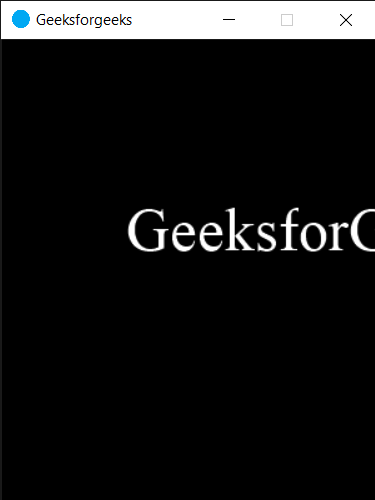# PYGLET – On Resize Event

In this article we will see how we can trigger on resize event in PYGLET module in python. Pyglet is easy to use but powerful library for developing visually rich GUI applications like games, multimedia etc. A window is a “heavyweight” object occupying operating system resources. Windows may appear as floating regions or can be set to fill an entire screen (fullscreen).This event get called when the window was resized. The window will have the GL context when this event is dispatched; there is no need to call switch_to in this handler. We can change the size of the window with the help of set_size method.

We can create a window with the help of command given below

`pyglet.window.Window(width, height, title)`

Below is the syntax of the on resize event, this method get called when this event is triggered

```@window.event
def on_resize(width, height):
print("Window Resized")```

Below is the implementation

## Python3

 `# importing pyglet module` `import` `pyglet` `import` `pyglet.window.key`   `# width of window` `width ``=` `500`   `# height of window` `height ``=` `500`   `# caption i.e title of the window` `title ``=` `"Geeksforgeeks"`   `# creating a window` `window ``=` `pyglet.window.Window(width, height, title)`   `# text ` `text ``=` `"GeeksforGeeks"`   `# creating a label with font = times roman` `# font size = 36` `# aligning it to the center` `label ``=` `pyglet.text.Label(text,` `                          ``font_name ``=``'Times New Roman'``,` `                          ``font_size ``=` `36``,` `                          ``x ``=` `window.width``/``/``2``, y ``=` `window.height``/``/``2``,` `                          ``anchor_x ``=``'center'``, anchor_y ``=``'center'``)`   `new_label ``=` `pyglet.text.Label(text,` `                          ``font_name ``=``'Times New Roman'``,` `                          ``font_size ``=` `10``,` `                          ``x ``=` `25``, y ``=` `25``)`   `# on draw event` `@window``.event` `def` `on_draw():    `   `    `  `    ``# clearing the window` `    ``window.clear()` `    `  `    ``# drawing the label on the window` `    ``label.draw()`   `    `  `# key press event    ` `@window``.event` `def` `on_key_press(symbol, modifier):`   `    ``# key "C" get press` `    ``if` `symbol ``=``=` `pyglet.window.key.C:` `        `  `        ``print``(``"Key C is pressed"``)`     `# on resize event` `@window``.event` `def` `on_resize(width, height):` `    `  `    ``# printing some message` `    ``print``(``"Window Resized"``)` `    ``print``(``"New Size : "``, end ``=` `"")` `    ``print``(width, height)` `    `    ` `    `# image for icon` `img ``=` `image ``=` `pyglet.resource.image(``"logo.png"``)`   `# setting image as icon` `window.set_icon(img)`   `# moving window` `window.set_size(``300``, ``400``)` `               `  `# start running the application` `pyglet.app.run()`

Output :```Window Resized
New Size : 300 400```

Whether you're preparing for your first job interview or aiming to upskill in this ever-evolving tech landscape, GeeksforGeeks Courses are your key to success. We provide top-quality content at affordable prices, all geared towards accelerating your growth in a time-bound manner. Join the millions we've already empowered, and we're here to do the same for you. Don't miss out - check it out now!

Previous
Next